Question

1. On Inverting Matrices, using Gauss-Jordan (a) Consider the following matrix A. If the inverse of...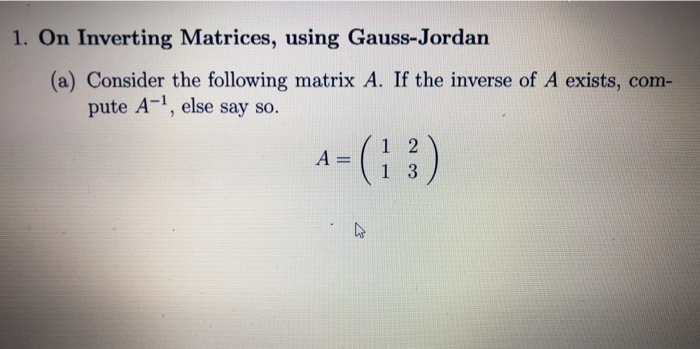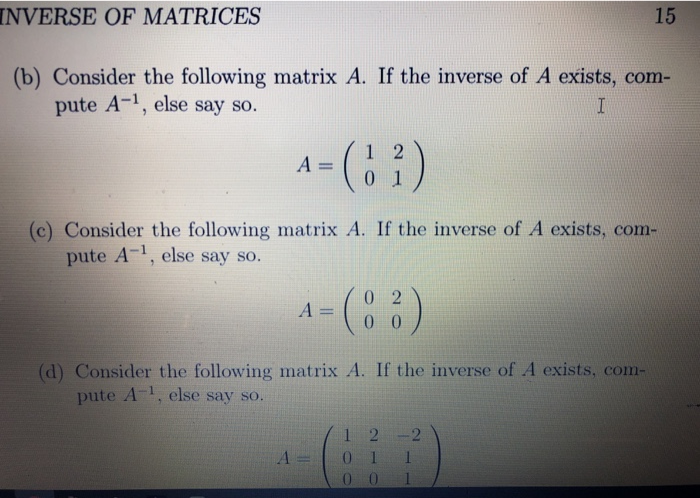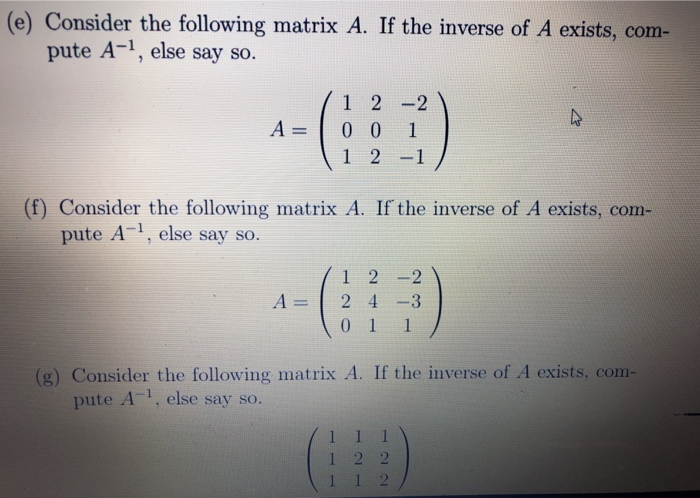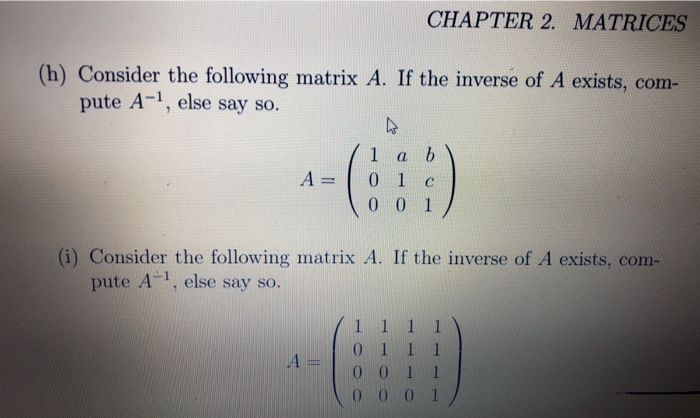1. On Inverting Matrices, using Gauss-Jordan (a) Consider the following matrix A. If the inverse of A exists, com- pute A-1, else say so. A 1 3
INVERSE OF MATRICES 15 (b) Consider the following matrix A. If the inverse of A exists, com- pute A-1, else say so. A-(3) 0 1 (c) Consider the following matrix A. If the inverse of A exists, com- pute A1, else say so. 0 2 (d) Consider the following matrix A. If the inverse of A exists, com- pute A, else say so. 1 2 2 0 0 l
(e) Consider the following matrix A. If the inverse of A exists, com- pute A-1, else say so. 1 2 -2 A 0 0 1 1 2 -1 (f) Consider the following matrix A. If the inverse of A exists, com- pute A-1, else say so. 1 2 2 A-124-3 011 (g) Consider the following matrix A. If the inverse of A exists, com- pute A-1, else say so.
CHAPTER 2. MATRICES (h) Consider the following matrix A. If the inverse of A exists, com- pute A-1, else say so. A=| 0 0 0 1 pute A1, else say so 0 0 1 1 乀0001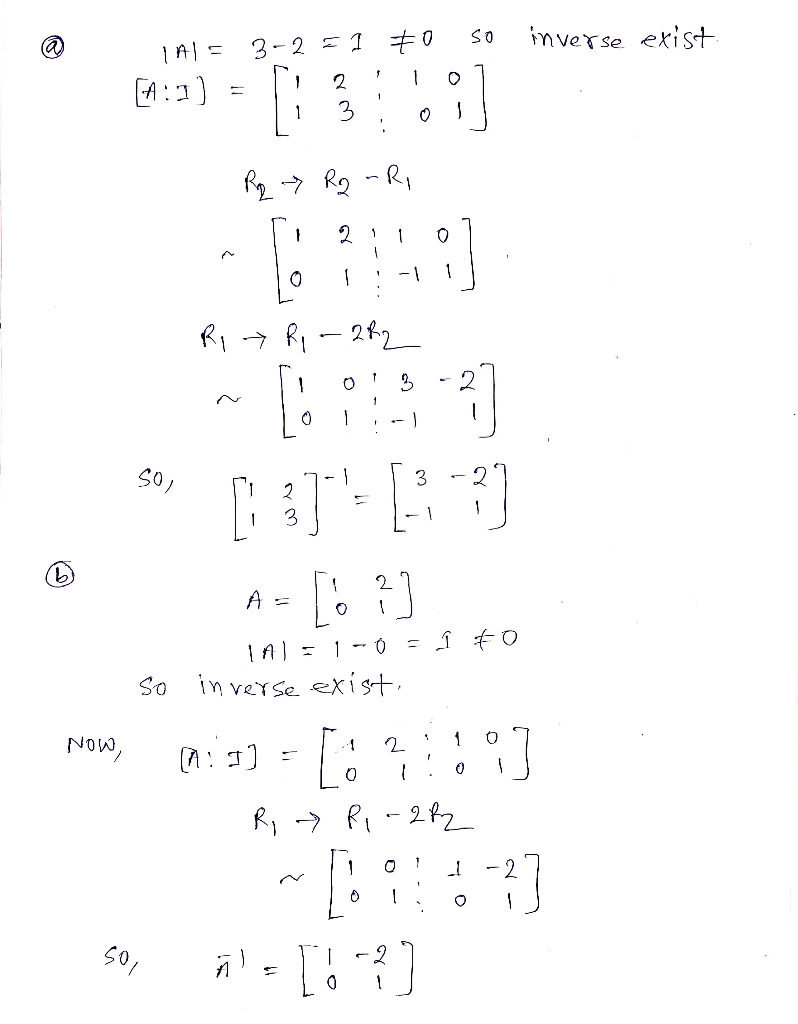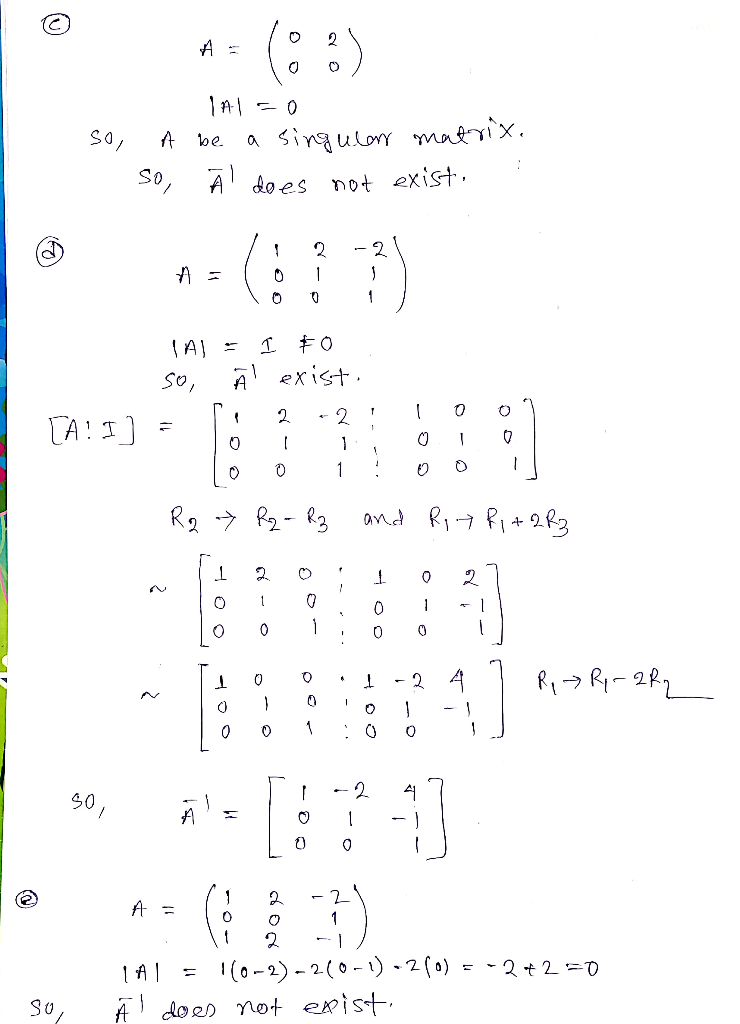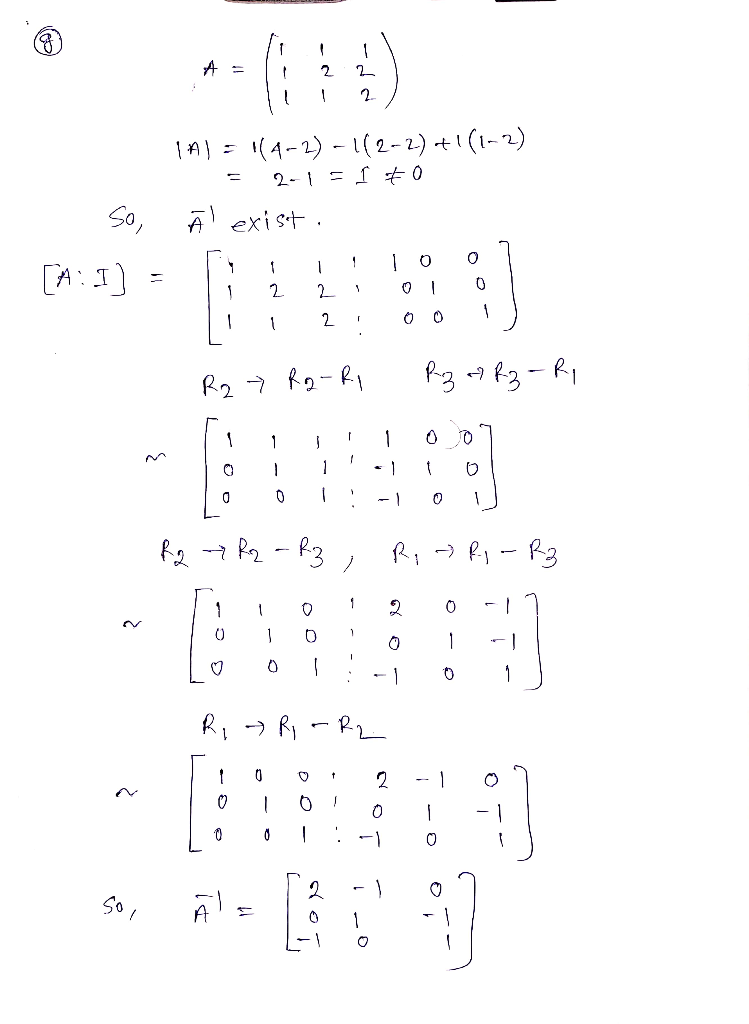Earn Coins

Coins can be redeemed for fabulous gifts.

Similar Homework Help Questions
• 4. Use elementary row operations (Gauss-Jordan method) to find the inverse of the matrix (if it...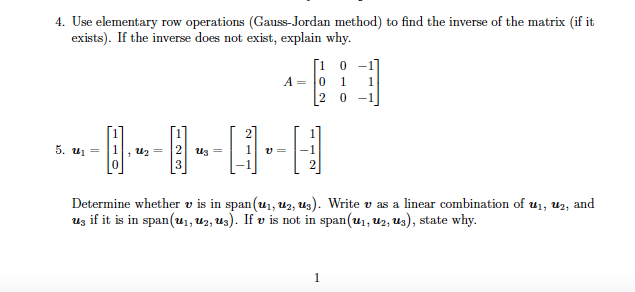4. Use elementary row operations (Gauss-Jordan method) to find the inverse of the matrix (if it exists). If the inverse does not exist, explain why. 1 0-1 A:0 1 2 0 -1 2us 0P 0 Determine whether v is in span(ui, u2, us). Write v as a linear combination of ui, u2, and us if it is in span(u1, u2, u3). If v is not in span(ui, u2, u3), state why. span(ui,u2,us). If v is not in span(ui,u^, us), state...

• Find the inverse of the following matrix A using Gauss-Jordan elimination (must show ALL steps): 20...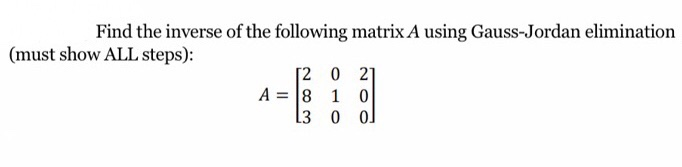Find the inverse of the following matrix A using Gauss-Jordan elimination (must show ALL steps): 20 21 A = 8 10 13 000

• Consider the following Gauss-Jordan reduction: Find... (1 point) Consider the following Gauss-Jordan reduction: TO -1 0...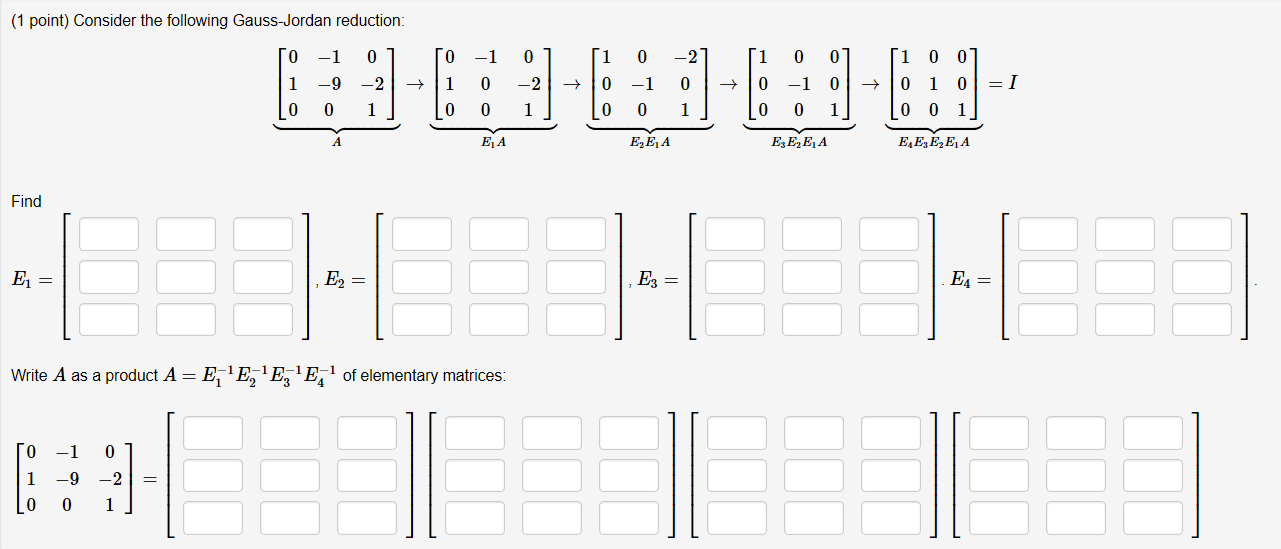Consider the following Gauss-Jordan reduction: Find... (1 point) Consider the following Gauss-Jordan reduction: TO -1 0 1 1 -9 -2 + Lo 0 1] 0 1 [o -1 O 0 0 1 -2 1] + [i 0 [o 0 -2 1 [ 100]  -1 0 → 0 -1 0 + 1010 = I 0 1 Lo 0 1] [o o 1] EZE, A EA E3E, E A E EZEE A Find E LEHEHEHE = 1 E3 = Write A...

• Find the inverse of the matrix below with the Gauss-Jordan method. (You can use decimal numbers...Find the inverse of the matrix below with the Gauss-Jordan method. (You can use decimal numbers or fractional numbers in the solution) (USE FOUR DIGITS AFTER THE DOT) 1 1 -1 0 2 -10 2 2 -1 1 -1 1 1 زرا 0

• Consider the following matrix: B= 5 2 - 2 3 (a) Use the Gauss-Jordan method to...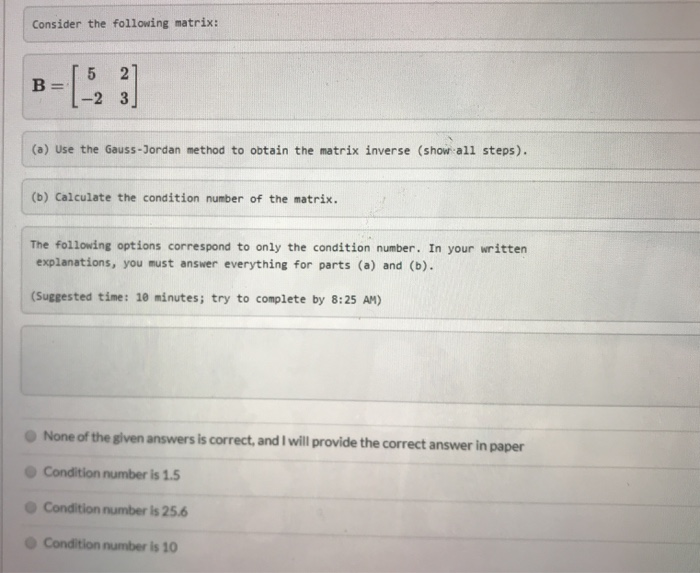Consider the following matrix: B= 5 2 - 2 3 (a) Use the Gauss-Jordan method to obtain the matrix inverse (show all steps). (b) Calculate the condition number of the matrix. The following options correspond to only the condition number. In your written explanations, you must answer everything for parts (a) and (b). (Suggested time: 10 minutes; try to complete by 8:25 AM) None of the given answers is correct, and I will provide the correct answer in paper Condition...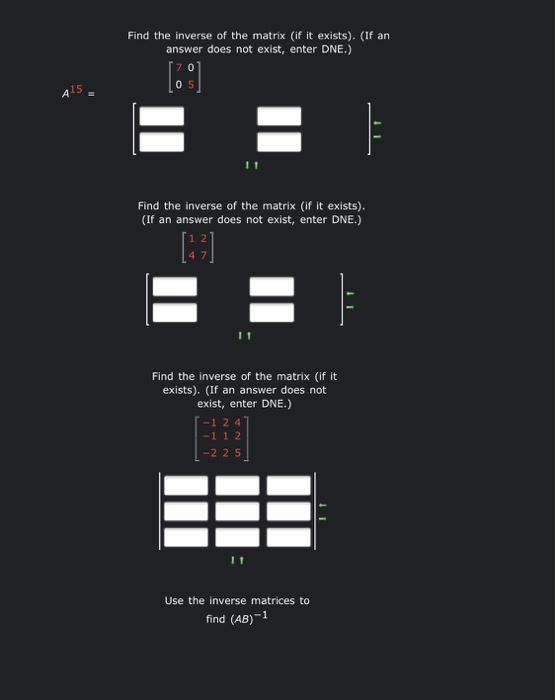3 part question about inverse of matrices. please help!! Find the inverse of the matrix (if it exists). (If an answer does not exist, enter DNE.)  05 415 E Find the inverse of the matrix (if it exists). (If an answer does not exist, enter DNE.) E = Find the inverse of the matrix (if it exists). (If an answer does not exist, enter DNE.) 1-1 2 4 -1 1 2 | -2 25 Use the inverse matrices to...

• 2. Inverse of a square matrix: Determine the inverse matrix [A™'] of the given square matrix...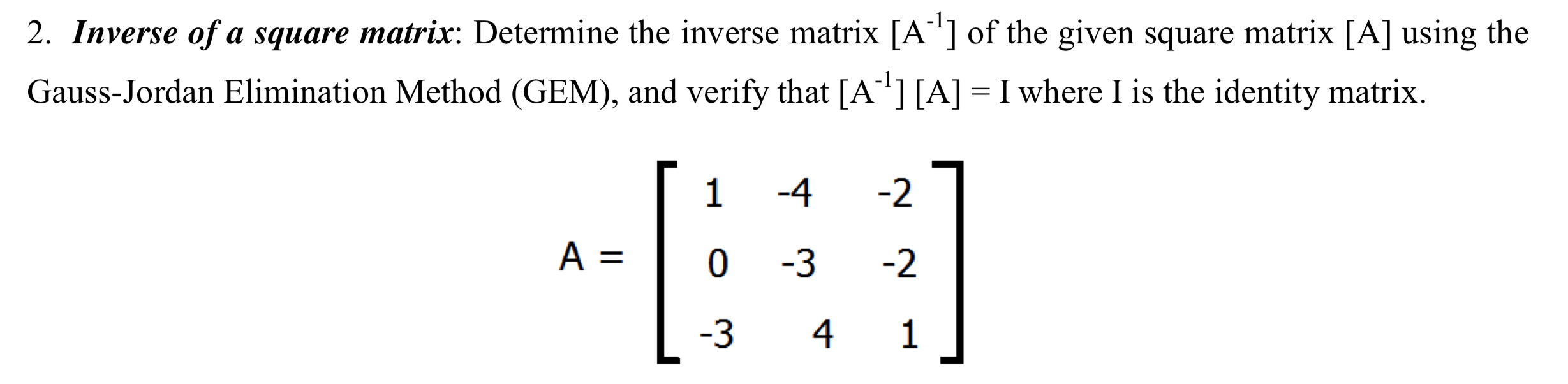2. Inverse of a square matrix: Determine the inverse matrix [A™'] of the given square matrix [A] using the Gauss-Jordan Elimination Method (GEM), and verify that [A-!] [A] = I where I is the identity matrix. A = [ 1 4 -27 0 -3 -2 | -3 4 1

• (1 point) Consider the following Gauss-Jordan reduction 1 0 0 200 → -2 0 01-11 00|→...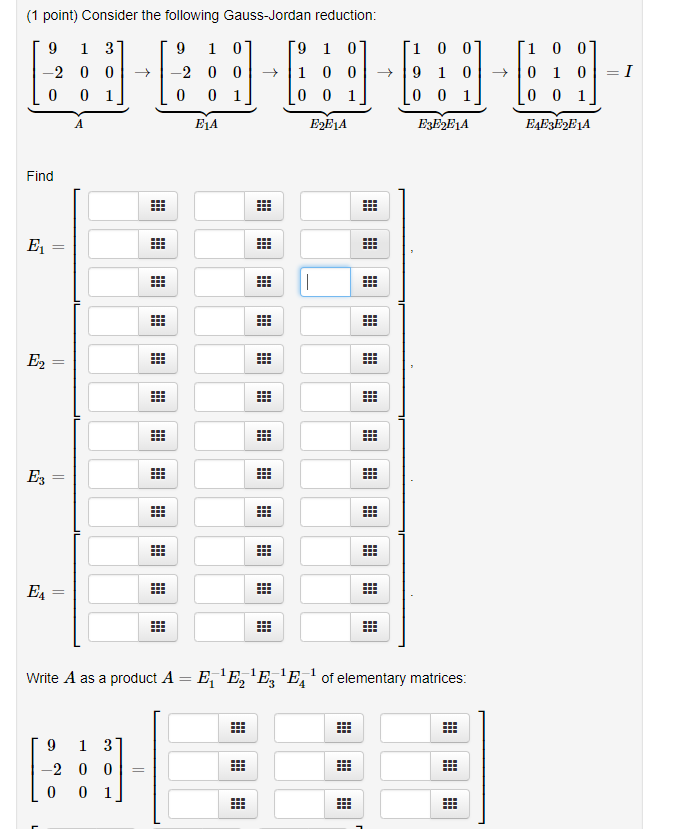(1 point) Consider the following Gauss-Jordan reduction 1 0 0 200 → -2 0 01-11 00|→ 9 1 01 .10 1 01-1 E1A E2E1A E4E3E2E1A Find E2 as a product AEE E of elementary matrices 2 0 0 Write A as a product A- E EE'Eof elementary matrices 1 2 3 4 91 31

• Algebra of matrices. 3. (a) If A is a square matrix, what does it mean to say that B is an inverse of A (b) Define AT....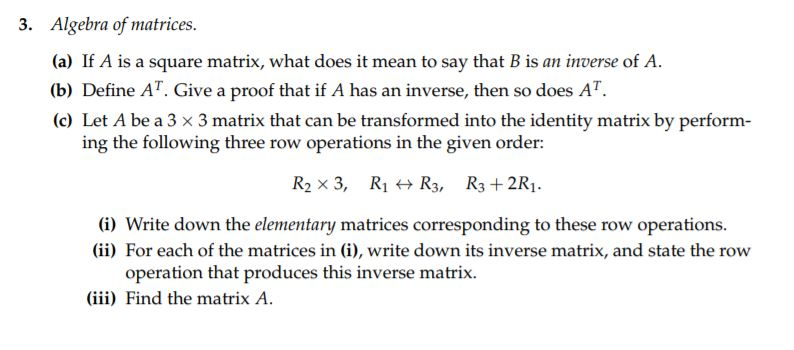Algebra of matrices. 3. (a) If A is a square matrix, what does it mean to say that B is an inverse of A (b) Define AT. Give a proof that if A has an inverse, then so does AT. (c) Let A be a 3 x 3 matrix that can be transformed into the identity matrix by perform ing the following three row operations in the given order: R2 x 3, Ri R3, R3+2R1 (i) Write down the elementary...

• Exercise 1 Consider the two matrices o 3 1 0 ) Say whether the following matrix...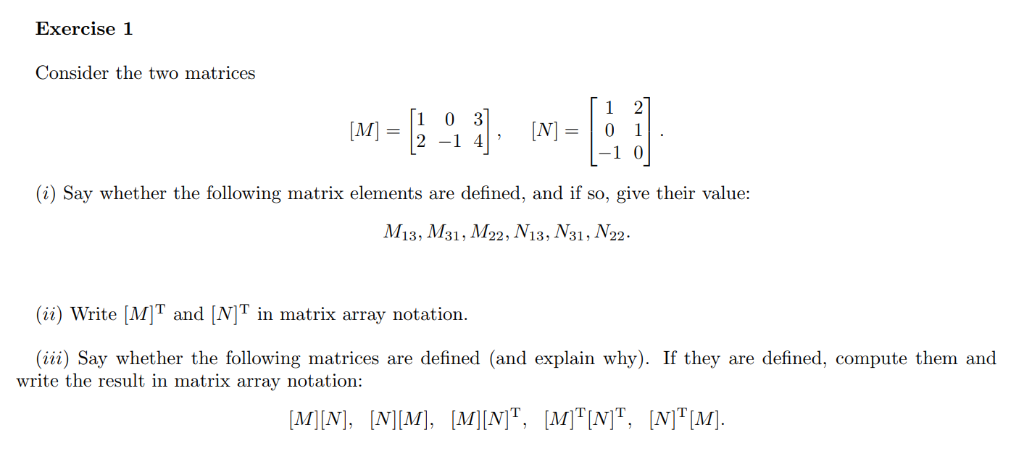Exercise 1 Consider the two matrices o 3 1 0 ) Say whether the following matrix elements are defined, and if so, give their value: 13, 1M31,M22, V13, V31, /V22 (i) Write MIT and [NI in matrix array notation. (iii) Say whether the following matrices are defined (and explain why). If they are defined, compute them and write the result in matrix array notation